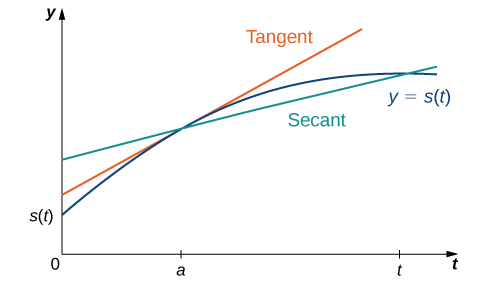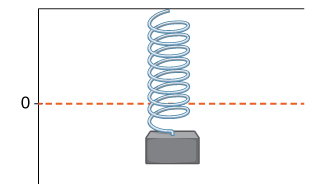# 3.1 Defining the derivative  (Page 4/10)

 Page 4 / 10
${v}_{\text{ave}}=\frac{s\left(t\right)-s\left(a\right)}{t-a}.$

As the values of $t$ approach $a,$ the values of ${v}_{\text{ave}}$ approach the value we call the instantaneous velocity    at $a.$ That is, instantaneous velocity at $a,$ denoted $v\left(a\right),$ is given by

$v\left(a\right)={s}^{\prime }\left(a\right)=\underset{t\to a}{\text{lim}}\frac{s\left(t\right)-s\left(a\right)}{t-a}.$

To better understand the relationship between average velocity and instantaneous velocity, see [link] . In this figure, the slope of the tangent line (shown in red) is the instantaneous velocity of the object at time $t=a$ whose position at time $t$ is given by the function $s\left(t\right).$ The slope of the secant line (shown in green) is the average velocity of the object over the time interval $\left[a,t\right].$The slope of the secant line is the average velocity over the interval [ a , t ] . The slope of the tangent line is the instantaneous velocity.

We can use [link] to calculate the instantaneous velocity, or we can estimate the velocity of a moving object by using a table of values. We can then confirm the estimate by using [link] .

## Estimating velocity

A lead weight on a spring is oscillating up and down. Its position at time $t$ with respect to a fixed horizontal line is given by $s\left(t\right)=\text{sin}\phantom{\rule{0.2em}{0ex}}t$ ( [link] ). Use a table of values to estimate $v\left(0\right).$ Check the estimate by using [link] .A lead weight suspended from a spring in vertical oscillatory motion.

We can estimate the instantaneous velocity at $t=0$ by computing a table of average velocities using values of $t$ approaching $0,$ as shown in [link] .

Average velocities using values of t Approaching 0
$t$ $\frac{\text{sin}\phantom{\rule{0.1em}{0ex}}t-\text{sin}\phantom{\rule{0.1em}{0ex}}0}{t-0}=\frac{\text{sin}\phantom{\rule{0.1em}{0ex}}t}{t}$
$-0.1$ $0.998334166$
$-0.01$ $0.9999833333$
$-0.001$ $0.999999833$
$0.001$ $0.999999833$
$0.01$ $0.9999833333$
$0.1$ $0.998334166$

From the table we see that the average velocity over the time interval $\left[-0.1,0\right]$ is $0.998334166,$ the average velocity over the time interval $\left[-0.01,0\right]$ is $0.9999833333,$ and so forth. Using this table of values, it appears that a good estimate is $v\left(0\right)=1.$

By using [link] , we can see that

$v\left(0\right)={s}^{\prime }\left(0\right)=\underset{t\to 0}{\text{lim}}\frac{\text{sin}\phantom{\rule{0.1em}{0ex}}t-\text{sin}\phantom{\rule{0.1em}{0ex}}0}{t-0}=\underset{t\to 0}{\text{lim}}\frac{\text{sin}\phantom{\rule{0.1em}{0ex}}t}{t}=1.$

Thus, in fact, $v\left(0\right)=1.$

A rock is dropped from a height of $64$ feet. Its height above ground at time $t$ seconds later is given by $s\left(t\right)=-16{t}^{2}+64,0\le t\le 2.$ Find its instantaneous velocity $1$ second after it is dropped, using [link] .

$-32$ ft/s

As we have seen throughout this section, the slope of a tangent line to a function and instantaneous velocity are related concepts. Each is calculated by computing a derivative and each measures the instantaneous rate of change of a function, or the rate of change of a function at any point along the function.

## Definition

The instantaneous rate of change    of a function $f\left(x\right)$ at a value $a$ is its derivative ${f}^{\prime }\left(a\right).$

## Chapter opener: estimating rate of change of velocity(credit: modification of work by Codex41, Flickr)

Reaching a top speed of $270.49$ mph, the Hennessey Venom GT is one of the fastest cars in the world. In tests it went from $0$ to $60$ mph in $3.05$ seconds, from $0\phantom{\rule{0.2em}{0ex}}\text{to}\phantom{\rule{0.2em}{0ex}}100$ mph in $5.88$ seconds, from $0\phantom{\rule{0.2em}{0ex}}\text{to}\phantom{\rule{0.2em}{0ex}}200$ mph in $14.51$ seconds, and from $0\phantom{\rule{0.2em}{0ex}}\text{to}\phantom{\rule{0.2em}{0ex}}229.9$ mph in $19.96$ seconds. Use this data to draw a conclusion about the rate of change of velocity (that is, its acceleration ) as it approaches $229.9$ mph. Does the rate at which the car is accelerating appear to be increasing, decreasing, or constant?

First observe that $60$ mph = $88$ ft/s, $100$ mph $\approx 146.67$ ft/s, $200$ mph $\approx 293.33$ ft/s, and $229.9$ mph $\approx 337.19$ ft/s. We can summarize the information in a table.

$v\left(t\right)$ At different values of t
$t$ $v\left(t\right)$
$0$ $0$
$3.05$ $88$
$5.88$ $147.67$
$14.51$ $293.33$
$19.96$ $337.19$

Now compute the average acceleration of the car in feet per second on intervals of the form $\left[t,19.96\right]$ as $t$ approaches $19.96,$ as shown in the following table.

Average acceleration
$t$ $\frac{v\left(t\right)-v\left(19.96\right)}{t-19.96}=\frac{v\left(t\right)-337.19}{t-19.96}$
$0.0$ $16.89$
$3.05$ $14.74$
$5.88$ $13.46$
$14.51$ $8.05$

The rate at which the car is accelerating is decreasing as its velocity approaches $229.9$ mph $\text{(}337.19$ ft/s).

#### Questions & Answers

find the domain and range of f(x)= 4x-7/x²-6x+8
Nick Reply
find the range of f(x)=(x+1)(x+4)
Jane Reply
-1, -4
Marcia
That's domain. The range is [-9/4,+infinity)
Jacob
If you're using calculus to find the range, you have to find the extrema through the first derivative test and then substitute the x-value for the extrema back into the original equation.
Jacob
Good morning,,, how are you
Harrieta Reply
d/dx{1/y - lny + X^3.Y^5}
mogomotsi Reply
How to identify domain and range
Umar Reply
hello
Akpevwe
He,,
Harrieta
hi
Dr
hello
velocity
I only talk to girls
Dr
women are smart then guys
Dr
Smarter
Adri
sorry
Dr
hi adri ana
Dr
:(
Shun
was up
Dr
hello
Adarsh
is it chatting app?.. I do not see any calculus here. lol
Adarsh
Find the arc length of the graph of f(x) = In (sinx) on the interval [Π/4, Π/2].
mukul Reply
Sand falling freely from a lorry form a conical shape whose height is always equal to one-third the radius of the base. a. How fast is the volume increasing when the radius of the base is (1m) and increasing at the rate of 1/4cm/sec Pls help me solve
ade
show that lim f(x) + lim g(x)=m+l
BARNABAS Reply
list the basic elementary differentials
Chio Reply
Differentiation and integration
Okikiola Reply
yes
Damien
proper definition of derivative
Syed Reply
the maximum rate of change of one variable with respect to another variable
Amdad
terms of an AP is 1/v and the vth term is 1/u show that the sum of uv terms is 1/2(uv+1)
Inembo Reply
what is calculus?
BISWAJIT Reply
calculus is math that studies the change in math, such as the rate and distance,
Tamarcus
what are the topics in calculus
Augustine
what is limit of a function?
Geoffrey Reply
what is x and how x=9.1 take?
Pravin Reply
what is f(x)
Inembo Reply
the function at x
Marc
also known as the y value so I could say y=2x or f(x)= 2x same thing just using functional notation your next question is what is dependent and independent variables. I am Dyslexic but know math and which is which confuses me. but one can vary the x value while y depends on which x you use. also
Marc
up domain and range
Marc
enjoy your work and good luck
Marc
I actually wanted to ask another questions on sets if u dont mind please?
Inembo
I have so many questions on set and I really love dis app I never believed u would reply
Inembo
Hmm go ahead and ask you got me curious too much conversation here
Adri
am sorry for disturbing I really want to know math that's why *I want to know the meaning of those symbols in sets* e.g n,U,A', etc pls I want to know it and how to solve its problems
Inembo
and how can i solve a question like dis *in a group of 40 students, 32 offer maths and 24 offer physics and 4 offer neither maths nor physics , how many offer both maths and physics*
Inembo
next questions what do dy mean by (A' n B^c)^c'
Inembo
The sets help you to define the function. The function is like a magic box where you put inside stuff(numbers or sets) and you get out the stuff but in different shapes (forms).
Adri
I dont understand what you wanna say by (A' n B^c)^c'
Adri
(A' n B (rise to the power of c)) all rise to the power of c
Inembo
Aaaahh
Adri
Ok so the set is formed by vectors and not numbers
Adri
A vector of length n
Adri
But you can make a set out of matrixes as well
Adri
I I don't even understand sets I wat to know d meaning of all d symbolsnon sets
Inembo
Wait what's your math level?
Adri
High-school?
Adri
yes
Inembo
am having big problem understanding sets more than other math topics
Inembo
So f:R->R means that the function takes real numbers and provides real numer. For ex. If f(x) =2x this means if you give to your function a real number like 2,it gives you also a real number 2times2=4
Adri
pls answer this question *in a group of 40 students, 32 offer maths and 24 offer physics and 4 offer neither maths nor physics , how many offer both maths and physics*
Inembo
If you have f:R^n->R^n you give to your function a vector of length n like (a1,a2,...an) where all a1,.. an are reals and gives you also a vector of length n... I don't know if i answering your question. Otherwise on YouTube you havr many videos where they explain it in a simple way
Adri
I would say 24
Adri
Offer both
Adri
Sorry 20
Adri
Actually you have 40 - 4 =36 who offer maths or physics or both.
Adri
I know its 20 but how to prove it
Inembo
You have 32+24=56who offer courses
Adri
56-36=20 who give both courses... I would say that
Adri
solution: In a question involving sets and Venn diagram, the sum of the members of set A + set B - the joint members of both set A and B + the members that are not in sets A or B = the total members of the set. In symbolic form n(A U B) = n(A) + n (B) - n (A and B) + n (A U B)'.
Mckenzie
In the case of sets A and B use the letters m and p to represent the sets and we have: n (M U P) = 40; n (M) = 24; n (P) = 32; n (M and P) = unknown; n (M U P)' = 4
Mckenzie
Now substitute the numerical values for the symbolic representation 40 = 24 + 32 - n(M and P) + 4 Now solve for the unknown using algebra: 40 = 24 + 32+ 4 - n(M and P) 40 = 60 - n(M and P) Add n(M and P), as well, subtract 40 from both sides of the equation to find the answer.
Mckenzie
40 - 40 + n(M and P) = 60 - 40 - n(M and P) + n(M and P) Solution: n(M and P) = 20
Mckenzie
thanks
Inembo
Simpler form: Add the sums of set M, set P and the complement of the union of sets M and P then subtract the number of students from the total.
Mckenzie
n(M and P) = (32 + 24 + 4) - 40 = 60 - 40 = 20
Mckenzie

### Read also:

#### Get the best Calculus volume 1 course in your pocket!

Source:  OpenStax, Calculus volume 1. OpenStax CNX. Feb 05, 2016 Download for free at http://cnx.org/content/col11964/1.2
Google Play and the Google Play logo are trademarks of Google Inc.

Notification Switch

Would you like to follow the 'Calculus volume 1' conversation and receive update notifications?ByByByBy